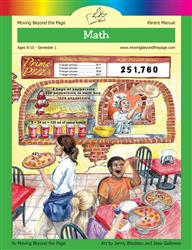Age 5-7 Reading Is Now Available!

# Common Core Alignment

CCSS.Math.Content.4.G.1 - Draw points, lines, line segments, rays, angles (right, acute, obtuse), and perpendicular and parallel lines. Identify these in two-dimensional figures.

## 5: MathUnit 3: Geometry
Lesson 1: Types of Lines
Lesson 11: Problem Solving
Lesson 12: Unit Test
Lesson 2: Points, Lines, Line Segments, Rays
Lesson 3: Angle Basics
Lesson 4: Measuring Angles
Lesson 5: Using a Protractor
Lesson 6: Playing with Angles
Lesson 7: Adding Angles
Final Project: My Geometry Town